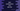# Constructors of a Dart list# Introduction :

Dart list comes with a couple of different constructors. We can create one empty list, a list with filled values, etc. using these constructors. In this tutorial, we will learn how to use different types of dart list constructors with examples.

## 1. ‘List’ constructor :

This constructor is defined as below :

``List([int length])``

We can create fixed length or growable length lists in dart. This constructor creates a list of fixed length if we provide the value of length. Else, it will create one growable length list.

For a fixed-length list, the length can’t be changed once created. For a growable list, it creates one with length 0. But we can also create one growable list with an initial length.

### Example :

``````main(List< string> args) {
var list1 = new List(5);
print("Size of list1 is \${list1.length}");

var list2 = new List();
print("Size of list2 is \${list2.length}");

var list3 = new List()..length = 3;
print("Size of list3 is \${list3.length}");
}``````

We have created three lists in this example. The first list list1 is a fixed length list of length 5. The second list list2 is a growable list of length 0 and the third list list3 is also a growable list of initial length 3.

## 2. ‘filled’ constructor :

filled constructor is defined as below :

``List.filled( int length, E e, { bool growable: false })``

It will create a list of size length and pre-fill all elements with e. You can also pass one more flag growable to this constructor. It will create one growable list if you pass true. The default value for growable is false.

``````main(List< string> args) {
var list1 = new List.filled(3, 4);
print(list1);

var list2 = new List.filled(2, 5, growable: true);
list2.length = 3;

print(list2);
}``````

It will print the below output :

``````[4, 4, 4]
[5, 5, null]``````

The first list list is a fixed-length list and list2 is a growable list. We can’t change the length of list1 like we did for list2.

## 3. ‘from’ constructor :

from constructor is defined as below :

``List.from(Iterable elements, {bool growable: true})``

This constructor creates one list from an iterable. It takes the iterable as its argument and creates one list. The second parameter growable defines if the final list should be growable or not. The default value is true.

``````main(List< string> args) {
var list1 = new List.filled(3, 4);

var list2 = new List.from(list1);
list2.length = 5;

print(list2);
}``````

It will print :

``[4, 4, 4, null, null]``

We have created one growable list list2 from the fixed-length list list1 with the same set of values.

## 4. ‘generate’ constructor :

generate constructor is defined as below :

``List.generate(int length, E generator( int index), {bool growable: true})``
``````main(List< string> args) {
var list = List.generate(5, (int index) => index % 2 == 0 ? 0 : 1, growable: false);
print(list);
}``````

This example creates a fixed-length list of size 5 with 0 for all even indices and 1 for all odd indices. It will print the below output :

``[0, 1, 0, 1, 0]``

## 5. ‘of’ constructor :

It is defined as below :

``List.of(Iterable itearble, {bool growable: true})``

It creates one list using an iterable. By default, it returns one growable list. If you want a fixed-length list, pass growable as false.

``````main(List< string> args) {
var n = new Iterable.generate(4, (i) => i);
var list = List.of(n, growable: false);

print(list);
}``````

It will print the below output :

``[0, 1, 2, 3]``

## 6. ‘unmodifiable’ constructor :

In dart, we can create one ‘unmodifiable’ list. We can’t change any length or elements of an ‘unmodifiable’ list. The constructor to create one unmodifiable list is defined as below :

``List.unmodifiable( Iterable i)``

It creates one ‘unmodifiable’ list containing all values of the iterable i. For example :

``````main(List< string> args) {
var n = new Iterable.generate(4, (i) => i*i);
var list = List.unmodifiable(n);

print(list);
}``````

It will print :

``[0, 1, 4, 9]``